Courses

# NCERT Solutions - Thermodynamics Class 11 Notes | EduRev

## JEE : NCERT Solutions - Thermodynamics Class 11 Notes | EduRev

The document NCERT Solutions - Thermodynamics Class 11 Notes | EduRev is a part of the JEE Course Physics For JEE.
All you need of JEE at this link: JEE

Ques 12.1: A geyser heats water flowing at the rate of 3.0 liters per minute from 27°C to 77°C. If the geyser operates on a gas burner, what is the rate of consumption of the fuel if its heat of combustion is 4.0 × 104 J/g.
Ans: Volume of water heated = 3.0 litre per minute Mass of water heated, m = 3000g per minute Increase in temperature,

ΔT - 77°C - 27°C = 50°C
Specific heat of water, c = 4.2 Jg-1°C-1
amount of heat used,  Q  =  mc ΔT
or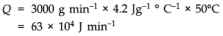Rate of combustion of fuel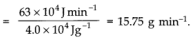Ques 12.2: What amount of heat must be supplied to 2.0 × 10–2 kg of nitrogen (at room temperature) to raise its temperature by 45 °C at constant pressure? (Molecular mass of N2 = 28; = 8.3 J mol–1 K–1.)
Ans12.2:
Here, mass of gas,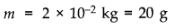rise in temperature,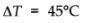Heat required.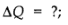Molecular mass, M = 28
Number of moles,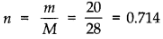As nitrogen is a diatomic gas, molar specific heat at constant pressure is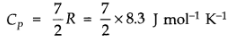As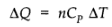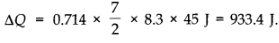Ques 12.3:Explain why
(a) Two bodies at different temperatures T1 and T2, if brought in thermal contact, do not necessarily settle to the mean temperature (T1 + T2)/2.
(b) The coolant in a chemical or a nuclear plant (i.e., the liquid used to prevent the different parts of a plant from getting too hot) should have high specific heat.
(c) Air pressure in a car tyre increases during driving.
(d) The climate of a harbour town is more temperate than that of a town in a desert at the same latitude.
Answer:
(a) In thermal contact, heat flows from the body at higher temperature to the body at lower temperature till temperatures become equal. The equilibrium temperature can be the mean temperature (T1 + T2 )/2 only when thermal capacities of the two bodies are equal.

(b) This is because heat absorbed by a substance is directly proportional to the specific heat of the substance.

(c) When car is driven, some work is being done on types in order to overcome dissipative forces of friction and air resistance etc. This work done is transformed into heat, due to which temperature of the car types increases.

(d) The climate of a harbour town is more temperate (neither too hot nor too cool) due to formation of sea breeze at day time and land breeze at night time as already explained.

Ques 12.4: A cylinder with a movable piston contains 3 moles of hydrogen at standard temperature and pressure. The walls of the cylinder are made of a heat insulator, and the piston is insulated by having a pile of sand on it. By what factor does the pressure of the gas increase if the gas is compressed to half its original volume?
Ans:
Here the process is adiabatic compression and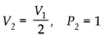atm and for hydrogen (a diatomic gas) γ = 1.4.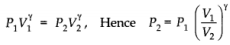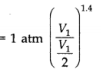⇒ = P2 (2)1.4 atm
= 2,64 atm,

Ques 12.5: In changing the state of a gas adiabatically from an equilibrium state to another equilibrium state B, an amount of work equal to 22.3 J is done on the system. If the gas is taken from state to via a process in which the net heat absorbed by the system is 9.35 cal, how much is the net work done by the system in the latter case? (Take 1 cal = 4.19 J)

Here, when the change is adiabatic,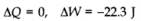If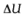is change in internal energy of. the system, then
as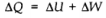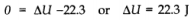Ans:  In the second case.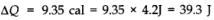ΔW = ?
As+ ΔW = ΔQ
ΔW = ΔQ -= 39.3 -22.3 - 17.0 J.

Ques 12.6: Two cylinders and of equal capacity are connected to each other via a stopcock. contains a gas at standard temperature and pressure. is completely evacuated. The entire system is thermally insulated. The stopcock is suddenly opened. Ansthe following:
(a) What is the final pressure of the gas in and B?
(b) What is the change in internal energy of the gas?
(c) What is the change in the temperature of the gas?
(d) Do the intermediate states of the system (before settling to the final equilibrium state) lie on its P-V-T surface?
Ans:
(a) Since the external temperature and initial temperature remain the same,

P2V2 = P1V1

But P1 = 1 atm, V1 = V, V2 = 2V and P2 - ?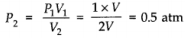(b) Since the temperature of the system remains unchanged, change in internal energy is zero.

(c) The system being thermally insulated, there is no change in temperature (because of free expansion)

(d) The expansion is a free expansion. Therefore, the intermediate states are non equilibrium states and the gas equation is not satisfied in these states. As a result, the gas can not return to an equilibrium state which lie on the P-V-T surface.

Ques 12.7: A steam engine delivers 5.4×10J of work per minute and services 3.6 × 10J of heat per minute from its boiler. What is the efficiency of the engine? How much heat is wasted per minute?
Ans 12.7:
Work done by the steam engine per minute, W = 5.4 × 108 J
Heat supplied from the boiler, H = 3.6 × 109 J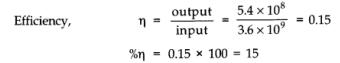Heat energy wasted/ minute

= Heat energy, absorbed/minute - Useful work done/minute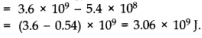Since    ΔQ =+ ΔW
∴ Change in internal energy,= ΔQ - ΔW
- 100 - 75 = 25 J/s.

Ques 12.8 A thermodynamic system is taken from an original state to an intermediate state by the linear process shown in Fig.Its volume is then reduced to the original value from E to F by an isobaric process. Calculate the total work done by the gas from D to E to F.
Answer:
As is clear from Fig.
Change in pressure, ΔP = EF = 5.0 - 2-0 “ 3-0 atm = 3.0 * 105 Nm-2
Change in volume, ΔV=DF = 600 - 300 = 300 cc
= 300 * 10-6 m3
Work done by the gas from D to E to F = area of ΔDEF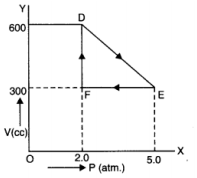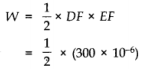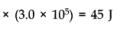Ques 12. 9 A refrigerator is to maintain eatables kept inside at 9 °C, if room temperature is 36 °C. Calculate the coefficient of performance.
Answer:
Here,  T1 = 36°C = (36 + 273) K = 309 K
T2 = 9°C = (9 + 273) K = 282 K

Coefficient of performance,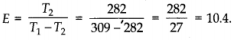Offer running on EduRev: Apply code STAYHOME200 to get INR 200 off on our premium plan EduRev Infinity!

## Physics For JEE

188 videos|346 docs|307 tests

,

,

,

,

,

,

,

,

,

,

,

,

,

,

,

,

,

,

,

,

,

;